# Finding Duplicates in a Sorted Array in C

## Finding Duplicates in a Sorted Array in C:

In this article, I am going to discuss Finding Duplicates in a Sorted Array in C Language with Examples. In our previous article, we have seen how to find multiple missing elements in a sorted array. In this article, we will see how to:

1. Find duplicate elements in a sorted Array
2. Count those duplicate elements.

Below is the example of the sorted array where we have taken duplicate elements: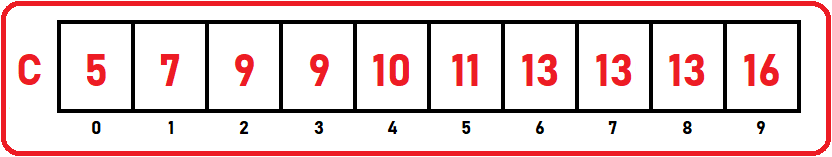First, let us develop some procedures for finding the duplicates then we will count those duplicates. For finding duplicates we should scan through this list. The total number of elements in the list is 10. So, we will take a counter ‘j’ and scan through the list. We have to take a variable ‘last_duplicate’ and initialize it with 0.

##### 0th Index: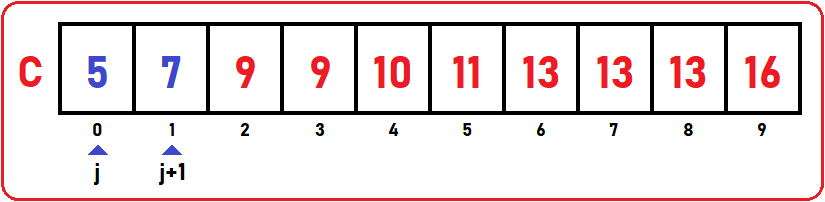Now j is on the 0th index, so being here we should check whether the next element is the same as this one. So, at C [j], we should check j+1. Is it the same? No, so move j to the next element.

last_duplicate = 0;

##### 1st Index: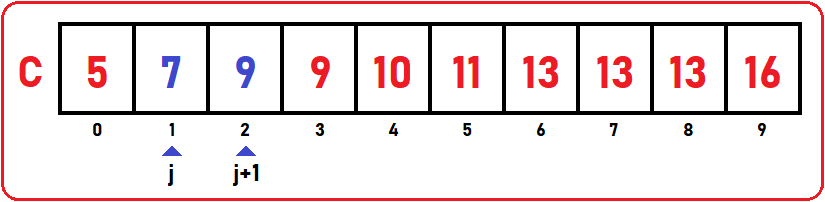Now j is on the 1st index, so we should check whether the next element is the same as this one. So at C [j], we should check j+1. Is it the same? No, so move j to the next element.

last_duplicate = 0;

##### 2nd Index: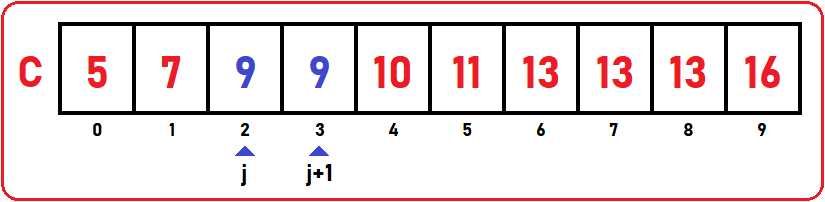Now j is on the 2nd index, so we should check whether the next element is the same as this one. So, at C [j], we should check j+1. Is it the same? Yes, the check is it equal to the last_duplicate variable? No, so print 9 on the screen and also we have to modify the duplicate variable to 9.

last_duplicate = 9;

As we can see, there are no duplicates till C  from C , so we directly jump to C :

##### 6th index: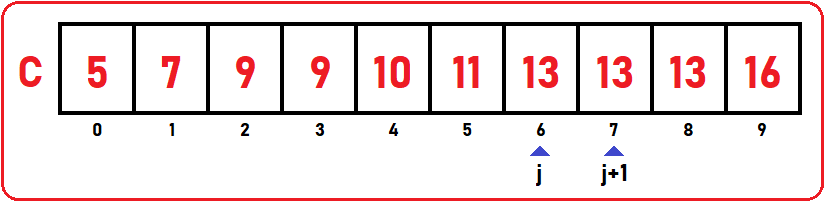Now j is on the 6th index, so we should check whether the next element is the same as this one. So, at C [j], we should check j+1. Is it the same? Yes, the check is it equal to the last_duplicate variable? No, so print 13 on the screen and modify the duplicate variable.

last_duplicate = 13;

##### 7th Index: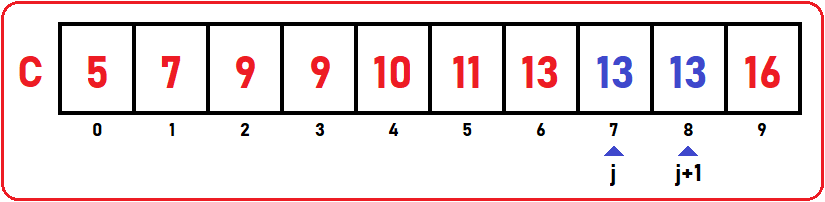Now j is on the 7th index, so we should check whether the next element is the same as this one. So at C [j], we should check j+1. Is it the same? Yes, but we have already printed 13 on the screen. We can’t print an element twice on the screen. This we have to care that if a number is duplicated more than one time then don’t print that element on the screen. So, here 13 is duplicated two times. Check if it is equal to the last_duplicate variable? Yes, then don’t print this and move j to the next element.

last_duplicate = 13;

We can clearly see that there are no more duplicates in the list, so we have left here. If more duplicates are present in the list, then the further procedure will work in the same manner as we discussed above.

##### Full Code in C Language:
```#include<stdio.h>
#include<stdlib.h>

struct List{
int C;
int size;
int length;
};

void Display(struct List list){
int i;
printf("Elements are\n");
for(i=0;i<list.length;i++)
printf("%d ",list.C[i]);

printf("\n\n");
}

void CheckDuplicate(struct List list){
int last_duplicate = 0;

printf("Duplicates are\n");
for(int i = 0; i < list.size; i++){
if(list.C[i] == list.C[i+1] && list.C[i] != last_duplicate){
printf("%d\n", list.C[i]);
last_duplicate = list.C[i];
}
}
}

int main()
{
struct List list_1 = {{5, 7, 9, 9, 10, 11, 13, 13, 13, 16},10,10};

Display(list_1);

CheckDuplicate(list_1);

return 0;
}

```
###### Output:Following is the code for printing duplicate elements with the number of times they appear:

##### Full Code in C language:
```#include<stdio.h>
#include<stdlib.h>

struct List{
int C;
int size;
int length;
};

void Display(struct List list){
int i;
printf("Elements are\n");
for(i=0;i<list.length;i++)
printf("%d ",list.C[i]);

printf("\n\n");
}

void CheckDuplicate(struct List list){
int j = 0;

printf("Duplicates are:\n");
for(int i = 0; i < list.size - 1; i++){
if(list.C[i] == list.C[i+1]){
j = i + 1;
while(list.C[j] == list.C[i])j++;
printf("%d is appearing %d time\n", list.C[i], j-i);
i = j-1;
}
}
}

int main()
{
struct List list_1 = {{5, 7, 9, 9, 10, 11, 13, 13, 13, 16},10,10};
Display(list_1);
CheckDuplicate(list_1);
return 0;
}
```
###### Output: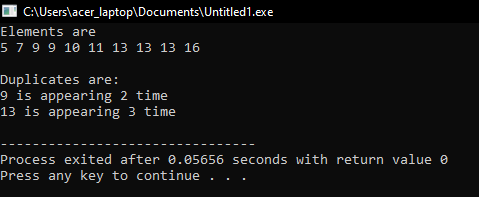In the next article, I am going to discuss Find Duplicate Elements in a Sorted Array using Hashing in C Language with Examples. Here, in this article, I try to explain How to Find Duplicates in a Sorted Array in C Language with Examples and I hope you enjoy this Finding Duplicates in a Sorted Array in C Language with Examples article.